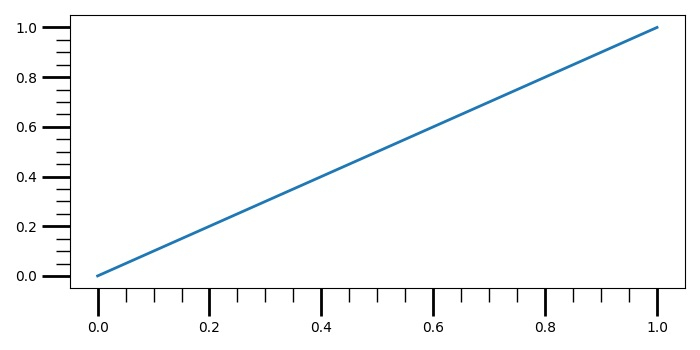# How to make longer subplot tick marks in Matplotlib?

To make longer subplot tick marks in matplotlib, we can use tick_params() method for minor and major ticks length and width.

## Steps

• Add a subplot to the current figure using subplot() method.

• Plot a range(2) value

• s for x and y data points.

• Turn the minor ticks of the colorbar ON without extruding into the "extend regions".

• Use tick_params for changing the appearance of ticks and tick labels.

• To display the figure, use show() method.

## Example

from matplotlib import pyplot as plt
plt.rcParams["figure.figsize"] = [7.00, 3.50]
plt.rcParams["figure.autolayout"] = True
ax1 = plt.subplot()
ax1.plot(range(2), range(2), linewidth=2)
ax1.minorticks_on()
ax1.tick_params('both', length=20, width=2, which='major')
ax1.tick_params('both', length=10, width=1, which='minor')
plt.show()

## Output